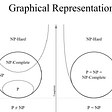# Prime numbers in complex domains are actually quite simple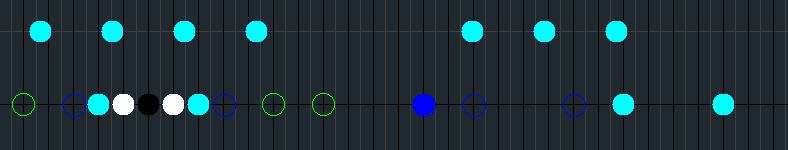Some primes in the ring of algebraic integers of Q(√−35). Hollow green dot at the left is −5, leftmost full cyan dot is −9/2 + (√−35)/2. This diagram was produced by version 0.95 of a Java program by Alonso del Arte.

On more than one occasion I’ve said that the so-called “complex” numbers are not at all complicated.

One such occasion was when I was showing my instructor and classmates at Integrate Detroit my Java program which draws diagrams of prime numbers in quadratic imaginary rings.

“What are we looking at?” asked Vaughn Walker, one of my classmates (we graduated a few weeks ago). It was a valid question that I did not properly address. Though I suppose I could at least have said it has to do with prime numbers in the complex plane.

It’s not something I can explain in 30 seconds or less. And even if I could explain it in such a short time, the explanation still requires some basic math background which the person hearing the explanation might not remember from high school math.

Or may have not been taught at all. I will now try to give a brief explanation of that background and then the explanation proper of what it is that my Java program draws diagrams of.

I can’t explain it in 30 seconds but maybe I can explain it in 30 minutes. If I explain it well, you’ll come away thinking that though this is an unfamiliar concept, it’s actually quite simple.

# Prime numbers

Hopefully everyone reading this knows what prime numbers are, and at least knows the basic definition. But even here there are a few unfamiliar nuances.

The domain of integers, …, −3, −2, −1, 0, 1, 2, 3, … is notated by mathematicians as Z (a bold capital Z, one can also use a blackboard bold Z).

A number p in Z, other than −1 or 1, is a prime number if it’s divisible only by −p, −1, 1 and p itself, and no other integers from Z. For example, 103 is prime since it’s divisible only by −103, −1, 1 and 103.

A stronger definition requires that if p divides a × b (or ab, as mathematicians prefer to write it), then either a or b must also be divisible by p (they may both be divisible by p, the important thing is that at least one of them is).

For example, 2 is a prime number, since for any possible choice of a and b such that ab is an even number we will find that either a or b is even as well, maybe both.

For example, −2 × −7 = 14, which is divisible by 2, and although −7 is not divisible by 2, −2 is. A perfectly easy and obvious fact.

To contrast that, we see that 4 is not a prime number, since, for example, although 2 × 14 = 28 is divisible by 4, neither 2 nor 14 is divisible by 4.

It is still required that p not be −1 or 1. Regardless of which of these definitions we go by, 1 is not a prime number. This is entirely because of those numbers’ intrinsic properties, and not for the usual idiotic reasons normally trotted out.

The thing is that −1 and 1 are units. Multiplying by units does not create distinct factorizations.

The prime factorization of −28, for example, can be expressed as 2² × (−7) or (−1) × 2² × 7 or (−2)² × −7 or whatever other combination will give us −28, but those can all be boiled down to one canonical form of our choosing.

This is the principle of unique factorization, and it means that Z is a unique factorization domain. The canonical form is a human invention, subject to change on our whims, but the underlying principle is eternal.

The first definition given here for prime numbers is actually a definition for irreducible numbers, which in some domains might not actually be prime by the second definition. At least in Z, it’s a distinction without a difference.

# Algebraic integers

You might vaguely remember solving simple quadratic equations in high school algebra. Equations like x² − 2 = 0.

You probably already know the answer to that one, but maybe in the context of homework or an exam you would have been expected to show the steps.

So maybe first you add 2 to both sides, turning the equation into x² = 2. Then, taking the square roots of both sides, you get x = √2, roughly 1.4.

Multiply by −1 to get the other solution, x = −√2, roughly −1.4. Then, remembering the fact that a negative number times a negative number is a positive number, we find that −1.4 × −1.4 = 1.96, which is 0.04 short of 2.

At this point I’m not too worried about numerical precision. If I need more decimal places, I’ll just punch it up in a calculator.

Here’s a slightly more difficult one: x² + 2x − 1 = 0. Adding 1 to both sides, we get x² + 2x = 1. And now please just humor me, let’s add 1 to both sides again, making our equation x² + 2x + 1 = 2.

This next step might seem a little magical: rewrite the left hand side as (x + 1)². You don’t have to take my word for it, you can verify it using FOIL (First product + Outer product + Inner product + Last product). Verify that (x + 1)(x + 1) = x² + x + x + 1 = x² + 2x + 1.

So our equation is now (x + 1)² = 2. Taking the square root of both sides, we get x + 1 = √2. Then subtract 1 from both sides to get the solution x = −1 + √2. The other solution is called the “conjugate”: x = −1 − √2.

The polynomials x² − 2 and x² + 2x − 1 are alike in that they can both be expressed as ax² + bx + c. In the former, b = 0 and c = −2, while in the latter, b = 2 and c = −1. But in both a = 1.

So if a, b and c are all integers from Z, then the corresponding numbers x are algebraic numbers with algebraic degree 2 at most (since 2 is the highest exponent in the polynomial).

And if a = 1, then x is an algebraic integer of algebraic degree 2 at most. This means that the numbers −√2, √2, −1 + √2 and −1 − √2 are not integers from Z but they are algebraic integers, with algebraic degree 2.

Furthermore, they are part of a domain of numbers called Z[√2], consisting of numbers of the form m + n√2, where m and n are both integers from Z.

Add or multiply any two algebraic integers from Z[√2] and the result is another algebraic integer from Z[√2]. Divide one algebraic integer from Z[√2] by another and the result may or may not also be in Z[√2], so the concept of divisibility applies in Z[√2] in much the same way it does in Z.

German mathematicians call domains like Z[√2] “rings,” highlighting the fact that they are closed under addition (understood to also mean subtraction) and multiplication, though not necessarily by division.

For example, √2 + (−1 + √2) = −1 + 2√2, which is a solution to x² + 2x − 7 = 0. And √2(−1 + √2) = 2 − √2, which is a solution to x² − 4x + 2 = 0.

The familiar integers of Z are also algebraic integers. For example, x = −7 is the only solution to x + 7 = 0. The algebraic degree is 1 (the exponent 1 is omitted but understood from the context).

And Z is contained within domains like Z[√2], in which case the numbers of Z are still of the form m + n√2, only that n = 0.

A number like 1/2 (one half) is an algebraic number but not an algebraic integer. We see x = 1/2 is a solution to 2x − 1, and so the coefficient attached to the x with the highest exponent is 2, not 1.

However, a number like 1/2 + (√5)/2 can be an algebraic integer. That one specifically is one of two solutions to x² − x − 1. This x is a special number called the golden ratio and usually denoted by the Greek letter φ (lowercase phi). But that’s a topic for another day.

So far you could have followed along with a typical scientific calculator without any problem. If you wanted to.

Though you may occasionally see a loss of machine precision, getting, for example, 0.99999999 or 1.00000001 when you expect exactly 1 (which is why the Java unit testing framework JUnit lets you specify an acceptable variance when testing that floating point numbers are equal).

For the next bunch of algebraic integers, though, you will need some workarounds if you want to follow along on a calculator like the one pictured above.

# Imaginary and complex numbers

If x² − 2 = 0 is easy to solve, x² + 2 = 0 should also be easy to solve, right? Subtract 2 from both sides to get x² = −2. Your calculator won’t self-destruct if you punch up √−2, but it will most likely lock up in an error state.

It seems no real number multiplied by itself will give a negative number. We saw earlier that −1.4 × −1.4 = +1.96, not −1.96. No increase in numerical precision will give us anything close to −2.

Often in algebra it is helpful to remember that √(ab) = (√a)(√b). You might remember this from high school algebra. Unfortunately it’s no help here: √−2 = (√−1)(√2), so we’re still dealing with the square root of a negative number.

Mathematicians dealt with problems of this sort four centuries ago. The solution that they came up with was to imagine that solutions do exist to equations like x² = −2.

They called the chief of these numbers they imagined the “imaginary” unit and assigned it the symbol i. Since i² = −1, it follows that √−2 = i√2, that is, roughly 1.4 multiplied by i.

Then the equation x² = −2 has two solutions, i√2 and −i√2, just as x² = 2 has two solutions, √2 and −√2. Numerically, i√2 is roughly 1.4142i and −i√2 is roughly −1.4142i.

And so they said that numbers like −1 and √2 are real, but numbers like i and √−2 are imaginary, even though they lead to a completely consistent system of numbers.

The psychological difficulty here is that real numbers measure solid things, but imaginary numbers measure things we still don’t fully understand, like the spin of subatomic particles.

This was all about a century before the great mathematician Carl Friedrich Gauss, who realized that the so-called imaginary numbers are just as real as the “real” numbers.

Now we have engineers and physicists to tell us that imaginary numbers are indeed real. And also Medium’s own Brett Berry, who explained it all very well in an article from a year ago.

However, the unfortunate terminology and notation have stuck, leading even people of great intelligence to think that imaginary and complex numbers are somehow beyond their comprehension.

As it turns out, just half-remembered high school algebra is enough to understand a good deal about imaginary and complex numbers.

A “complex” number is simply a number with both a “real” part and an “imaginary” part. The imaginary part is added to the real part, or vice-versa.

Algebraically, if m and n represent “real” numbers, then ni (meaning n multiplied by the imaginary unit i) represents a purely “imaginary” number and m + ni represents a “complex” number.

For complex numbers, the example I’ll use is a solution to the equation x² + 2x + 3 = 0. Just as before, we switch the “c” term to the other side: x² + 2x = −3.

Now as before, indulge me in adding 1 to both sides: x² + 2x + 1 = −2. This enables us to perform the same “magical” rewriting as before: (x + 1)² = −2. Taking the square root of both sides and subtracting 1 from both sides gives us one solution: x = −1 + √−2.

The other solution is, just as before, the conjugate, namely x = −1 − √−2. In both of these solutions, the real part is −1. In one solution, the imaginary part is √−2 or i√2, in the other solution the imaginary part is −√−2 or −i√2.

Just as 1/2 is an algebraic number but not an algebraic integer, i/2 is also an algebraic number but not an algebraic integer. The latter number is a solution to the equation 4x² + 1 = 0.

But −1/2 + (√−3)/2 is a solution to x² + x + 1 = 0. It is also a solution to x³ − 1 = 0 (an algebraic integer of a particular degree can be a solution to equations of higher algebraic degree), and as such it is a special number sometimes denoted by the Greek letter ω (lowercase omega, not be confused with w).

# Irreducible does not always mean prime

A number in Z[√2] is said to be divisible by another number in Z[√2] if the result of the division is also a number in Z[√2]. Likewise, a number in Z[√−2] is said to be divisible by another number in Z[√−2] if the result of the division is also a number in Z[√−2].

This means that numbers that are prime in Z are not necessarily prime in Z[√2], Z[√−2] or other domains of algebraic integers.

In particular, given x being a solution to x² + bx + c = 0 with c a prime in Z, it turns out that c is divisible by x in the relevant quadratic integer ring.

For example, in Z[√2], we see that 2 is divisible by √2, since 2 divided by √2 is √2. Similarly, in Z[√−2], we see that 2 is divisible by √−2, since 2 divided by √−2 is −√−2.

So in both domains, 2 is neither irreducible nor prime. Both √2 and √−2 are prime in their respective domains, as are numbers like 1 + √−2 and 3 − √2 (the former corresponds to x² − 2x + 3 and the integer 3, the latter to x² − 6x + 7 and the integer 7).

Both Z[√2] and Z[√−2] are unique factorization domains, and also principal ideal domains. The concept of “ideals” is also a simple concept that would take me more than a half hour to explain.

Suffice it to say here that it means that in these two domains all prime numbers are irreducible and all irreducible numbers are also prime. Just like in Z.

But in the vast majority of imaginary quadratic integer rings, several numbers have more than one distinct factorization. In fact, Z[√−2] is in a distinct minority, one of only nine rings.

As for Z[√2] among the purely real rings, that’s an open question I won’t get into here.

The most famous example of multiple distinct factorizations in an imaginary quadratic integer ring is 6 in Z[√−5], where we have (1 − √−5)(1 + √−5) = 6. But 2 and 3 are also irreducible in Z[√−5], and it is also true that 2 × 3 = 6.

We verify that 1 + √−5 is not divisible by either 2 or 3 in this ring: the minimal polynomial for 1/2 + (√−5)/2 is 2x² − 2x + 3, and the minimal polynomial for 1/3 + (√−5)/3 is 3x² − 2x + 2.

This is just as 6 is not divisible by 4 in Z. But in Z, both 4 and 6 are divisible by 2, and 6 is divisible by 3.

# Drawing the diagrams

From high school you probably remember the real number line. It is almost always horizontal, often with 0 at the center, with the negative numbers to the left of 0 and the positive numbers to the right.

Generally the real number line is shown with 0 at the center. We might see on the line, for example, −3, −2, −1, 0, 1, 2, 3. The imaginary number line can also be shown with 0 at the center. For example, −3i, −2i, −i, 0, i, 2i, 3i.

Since 0 is both purely real and purely imaginary, it only makes sense for it to be the juncture of the real and imaginary number lines. And to join them as perpendicular lines, so that 1 is at 90 degrees from i.

Then multiplication can be seen as rotation in the complex plane. Multiply by i to move 90 degrees. Multiply by i again to move another 90 degrees. Multiply by −1 to move 180 degrees. And multiply by 1 to move 360 degrees and end back where you started.

We can now locate purely imaginary numbers on the imaginary number line in the same way that we locate purely real numbers on the real number line. Here for example is √−2:

In the diagram above, I clicked with a mouse where I thought the dot for √−2 should go. Even allowing for some imprecision, it does not look right, it looks more like 1.3 than 1.4. It needs to be nudged up slightly.

Where do “complex” numbers, those numbers with nonzero real part and nonzero imaginary part, fit into all this? Easy: at the intersection of perpendicular lines drawn from the appropriate points on the real and imaginary axes.

For example, here’s how we locate 1 + √−2: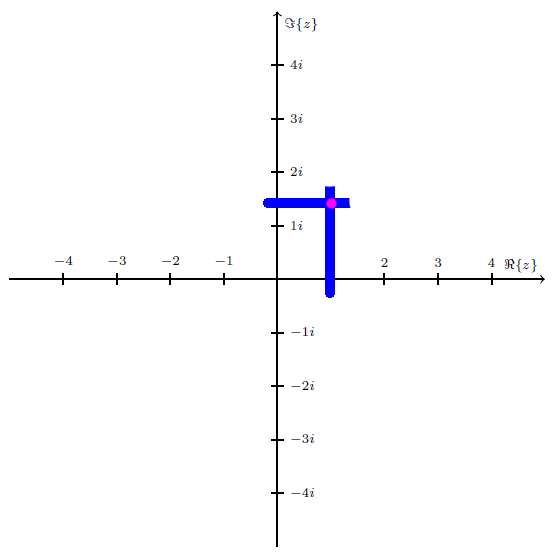The magenta dot gives the approximate location of 1 + √−2.

I’m fairly confident of where I placed the blue vertical line emanating from 1, but my placement of the blue line for √−2 fell a bit short of the mark.

Both of the last two preceding diagrams were created in Photoshop Elements based on the PDF of a TeX file. Most of the elements were placed by the computer, but both diagrams include “manually” placed elements.

It would be more reliably precise to draw a grid in which the vertical lines are spaced one unit apart and the horizontal lines are spaced √2 units apart. If our unit is 40 pixels, then √2 units can be 56 or 57 pixels (no display can really do 56.5685424949238 pixels).

And then I would click in dots for the units and dots for the prime numbers, one by one, by hand?

I actually did that for Z[i], Z[√−2] and Z[ω]. As I proceeded with those tasks, it became clear to me that I was under-utilizing my computer’s number-crunching power.

# Programming the computer to draw the diagrams

The obvious choice for the program was at first Wolfram Mathematica. After all, that’s what they used for the Wolfram MathWorld pages about Gaussian primes (primes in Z[i]) and Eisenstein primes (primes in Z[ω]).

And surely I could program Mathematica to draw more colorful diagrams than the ones on the MathWorld pages. But the biggest problem for me was that I have never actually had Wolfram Mathematica on my home computer.

Java, on the other hand, can be installed on almost any computer (though some caveats may loom on the horizon). The problem is that it has no innate facility for dealing with complex numbers.

C#, like FORTRAN, does come built in with support for complex numbers. But the numbers are represented “numerically” rather than “algebraically.” This could potentially mean that, for example, we get 2.99999999 + 0.00000001i when we expect 3 precisely.

So I decided to create a hierarchy of objects in Java to represent these numbers “symbolically,” kind of like how Mathematica does it.

So 1 + √−2 is not 1.00000000 + 1.41421356i, but an object with a “regular” part equal to the integer 1, and a “surd” part also equal to the integer 1, which is multiplied by the square root of −2.

The computations are done on the “fields” of the algebraic integer objects (here I mean “field” in the programming sense, not the mathematical sense), and conversion to pairs of floating point numbers occurs only when absolutely necessary.

As for the actual drawing of the diagrams, I realized I didn’t need to re-invent the wheel, though, for some reason I can’t quite explain now, I decided I would use pure Java AWT, no Java Swing.

After dealing with some unexpected problems and ugly kludges, I decided to use Java Swing after all. Pixar may not think much of Swing, but for my purpose, it’s more than adequate.

Where it might take me hours or days to draw one of these diagrams, my Java program can draw them in seconds, or milliseconds more likely than not. Some are more interesting than others, but it certainly doesn’t hurt to spend a few CPU clock cycles on one of these.

Here’s an example of Z[√−5], at 22 pixels per unit interval and a dot diameter of about that much.

The black dot at the center is 0. For now the program can only draw zero-centered diagrams. The units −1 and 1 are white dots to the left and right of 0.

The hollow green dots are −2 and 2, which bear a special relationship to −√−5 and √−5 (the full green dots above and below 0). The full green dots to the left and right of 0 are −5 and 5.

The hollow blue dots on the real number line (you may or may not be able to see them clearly in the diagram above) are numbers that are prime in Z, but in Z[√−5] they are irreducible but not prime. Like 7, since it divides 14 but not 3 − √−5 nor 3 + √−5, which are both solutions to x² − 6x + 14 = 0.

And the full cyan dots are numbers that are both irreducible and prime in this domain. With the program, you can zoom all the way out to 2 pixels per unit interval.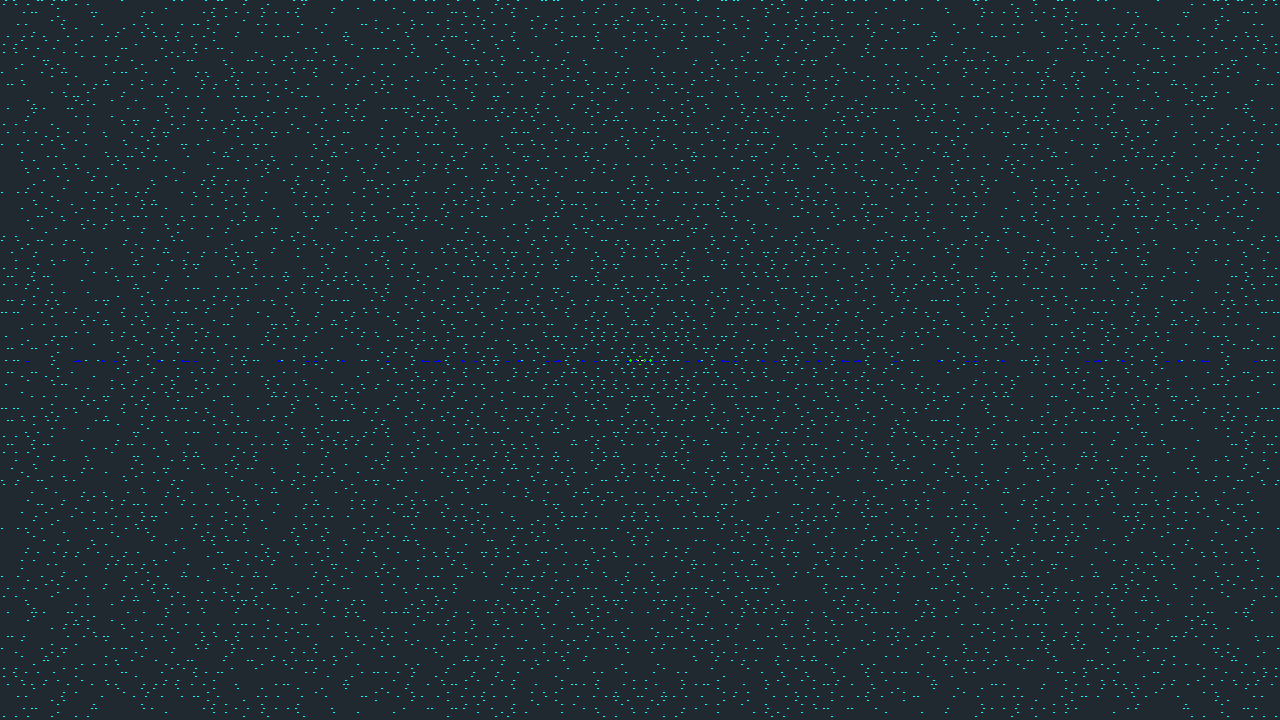Diagram of primes in Z[√−5], zoomed out to 2 pixels per unit interval.

If you want to see the diagram for, say, Z[√−10], in the program you can press Control-D or Command-D and enter in that number, −10 (you can also enter 10 and it will quietly change it to −10).

Here’s an interesting sequence to explore with the program: −7, −11, −19, −43, −67, −163:Diagram of primes in the ring of algebraic integers of Q(√−7).

Not really seeing anything so far, right?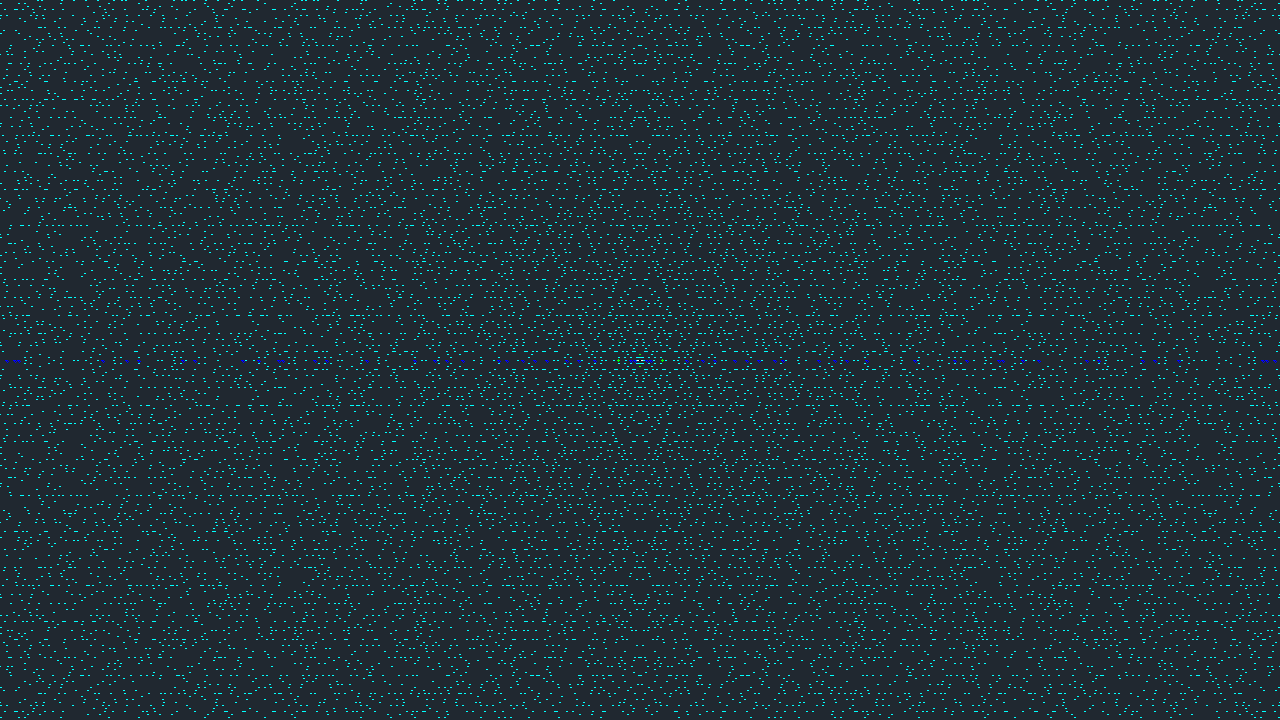Diagram of primes in the ring of algebraic integers of Q(√−11).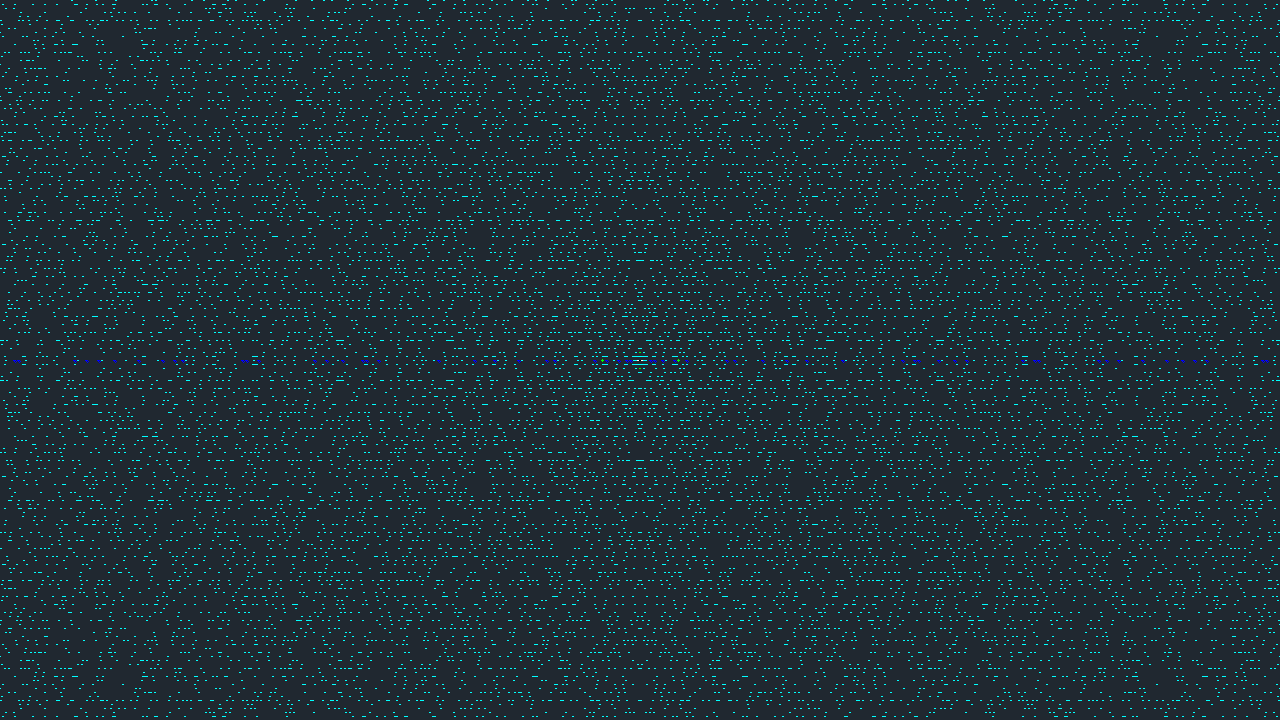Diagram of primes in the ring of algebraic integers of Q(√−19).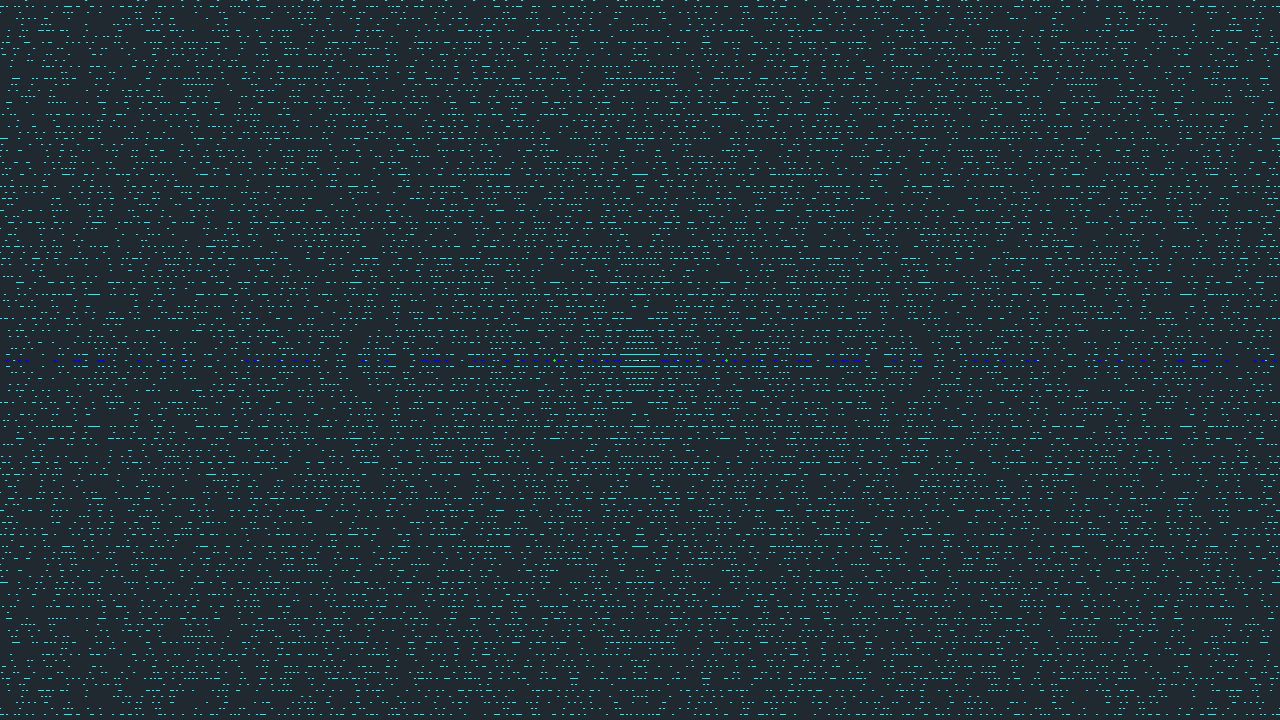Diagram of primes in the ring of algebraic integers of Q(√−43).

Wait, there’s something…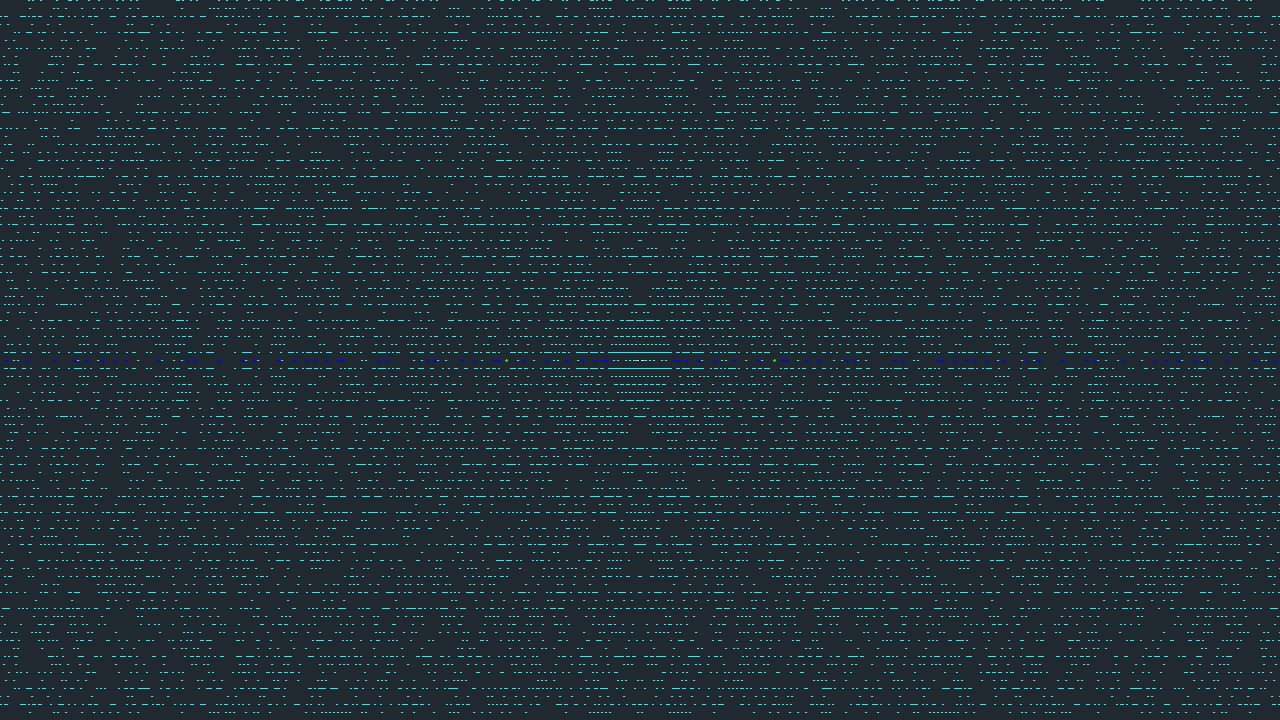Diagram of primes in the ring of algebraic integers of Q(√−67).

It’s like there’s this pasta shell (like one of those you can fill with ricotta cheese) in the middle of the diagram, but instead of it having the fill slit length-wise, the fill slit is width-wise.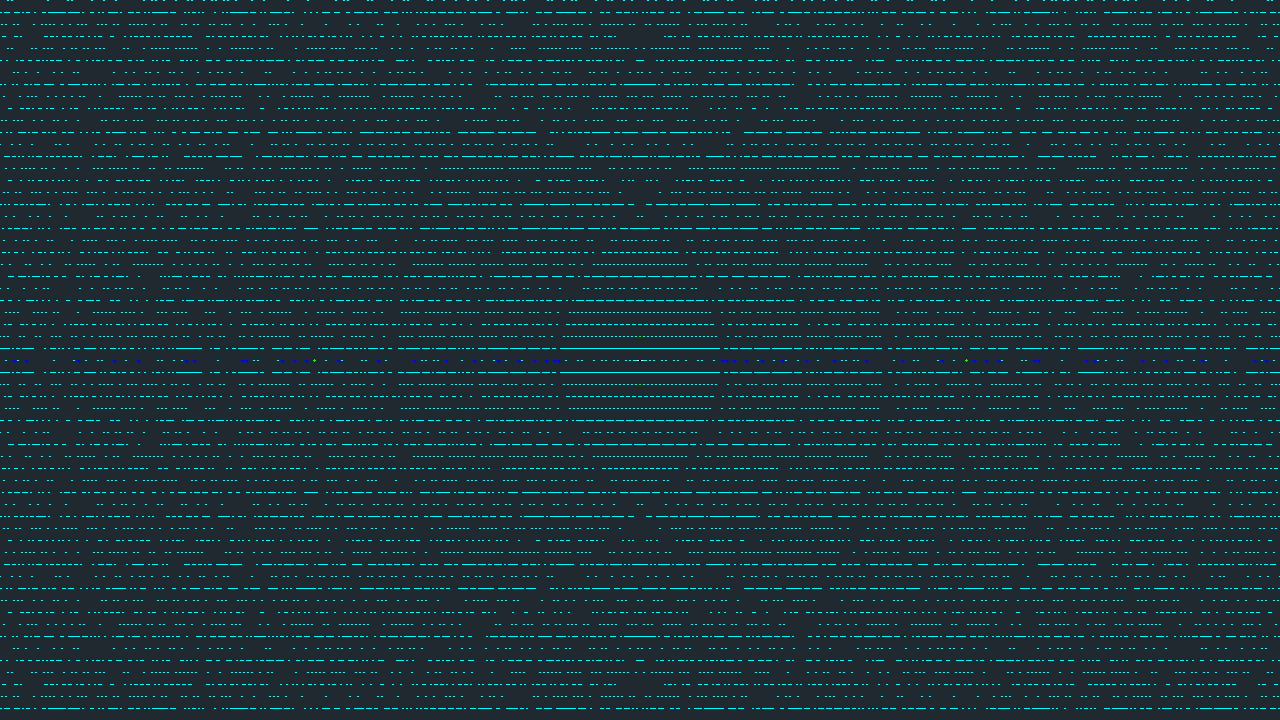Diagram of primes in the ring of algebraic integers of Q(√−163).

One person I showed this to called these “Star Wars dots.” But since I don’t have licensing from Disney, I guess I can’t call it that.

That’s the last of them like that. Maybe the great German mathematician Carl Friedrich Gauss saw this diagram in his mind two centuries ago, though likely with different colors, but as far as I know he never drew it nor even sketched it.

There are other negative numbers which produce diagrams that are interesting for other reasons.

The executable JAR for the program is barely 100KB, but it can produce megabytes’ worth of diagrams. I have seen a lot of these diagrams, but hardly all of them. I certainly hope other people use my program to find interesting diagrams I may have overlooked.

I envision this project eventually being worked on by a whole team, maybe by Version 3.0 or even 2.0. But Version 1.0 will be all me, except for two or three things I’ve copied off the Web.

All the source code and unit tests are available from my GitHub page. There you will also find an executable JAR file (Version 0.97, or it could be 0.98 or 0.99 if it’s not 1.0 yet) as well as some sample outputs.

This project did not start out as a test-driven development project, but I have gradually changed it over to that programming philosophy. Each new feature I add to the program (except those that depend on AWT or Swing) starts out with a stub, then a test and then the actual feature.

Eventually I’ll add the capability to the program to draw diagrams of prime numbers in domains of purely real numbers. Those turn out to be considerably more complicated than the domains of “complex” numbers.

Here’s a taste of Z[√2], in a diagram I made by clicking around in Photoshop Elements:

I can’t vouch for the precision of this diagram. There are no complex numbers here, but there certainly are complicated issues to deal with when I get around to writing the program.

It’s much easier with the complex numbers of a given imaginary domain because the numbers are neatly spaced throughout the complex plane, instead of clustered on the real number line in a seemingly random pattern.

--

--

--

## More from Alonso Del Arte

is a composer and photographer from Detroit, Michigan. He has been working on a Java program to display certain mathematical diagrams.

Love podcasts or audiobooks? Learn on the go with our new app.

## Through The Looking Glass: An Alternative Perspective of Mathematics Through Storytelling## Significance of the derivative## Decomposing Signal Using Empirical Mode Decomposition — Algorithm Explanation for Dummy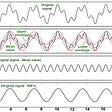## Mutually Exclusive And Independent Events## Infinite Powers: The Story Of Calculus By Steven Strogatz — Review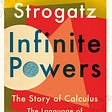## Orbiting stars and origin of our universe## Angry Professor## Alonso Del Arte

is a composer and photographer from Detroit, Michigan. He has been working on a Java program to display certain mathematical diagrams.

## function (hereWeGo) {## A Few Technical Notes on Test Functions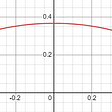## Complexity Theory - Part 2